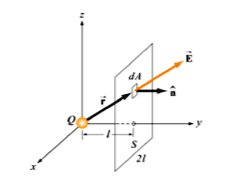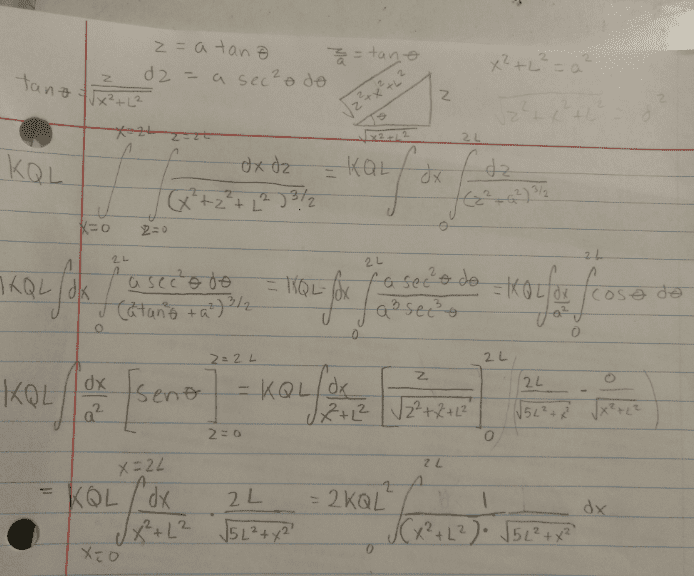# Gauss' Law problem: determine the electric flow through a square surface due to a nearby charge

## Homework Statement

determine the electric flow through a square surface of side 2l due to a load + Q located at a perpendicular distance l from the center of the plane

I really don't know how to answer this question .i need help guys
Thanks

## The Attempt at a Solution

I ended up with a doble integral ,which i had to evaluate in order to calculate the flow of electric fiele in the whole square

Last edited:

gneill
Mentor
Can you share the details of the the work you've done so far? Is there a figure that accompanies the problem that you can upload?this would be the image ,and i tried this:
im new to this kind of things also English is not my principal language

#### Attachments

Chandra Prayaga
You may not need to do an integration for this. Take advantage of the symmetry

•gneill
You may not need to do an integration for this. Take advantage of the symmetry
Should i just apply Gauss law And multiply by 1/6 ?

gneill
Mentor
Should i just apply Gauss law And multiply by 1/6 ?
If you can assume that the Y-axis passes through the center of the square so that symmetry is preserved, then yes.

Chandra Prayaga
The question will be, what is the closed surface on which you are applying Gauss's law, before dividing by 1/6?

The question will be, what is the closed surface on which you are applying Gauss's law, before dividing by 1/6?
A cube ,but i don't know if it's as easy as that

gneill
Mentor
A cube ,but i don't know if it's as easy as that
Actually, it is as easy as that! (With the assumption described in post #6).

Chandra Prayaga